### Introduction to Elliptic Curves

by

Elliptic curves are a special kind of algebraic curves which have a very rich arithmetical structure.

There are several fancy ways of defining them. but for our purposes we can just define them as the set of points satisfying a polynomial equation of a certain form. To be

specific, consider an equation of the form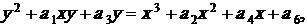where the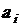are integers (There is a reason for the strange choice of indices on the, but we won't go into it here). we want to consider the set of points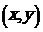which satisfy

this equation.

To make things easier, let us focus on the special case in which the equation is of the form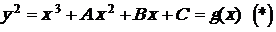with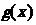a cubic polynomial (in other words, we're assuming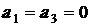). In this case (*), it's very easy to determine when there can be singular points, and even what

sort of singular points they will be. If we put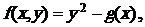Then we have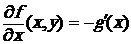and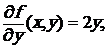we know, the curve will be smooth if there are no common solutions of the equations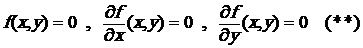Attention. we know, from elementary analysis, that an equation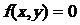defines a smooth curve exactly when there are no points on the curve at which both partial

derivatives of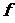vanish.

in other  words, the curve will be smooth if there are no common solutions of the equations (**).

And the condition for a point to be "bad" be comes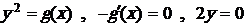which boils down to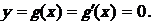In other words, a point will be "bad" exactly when its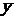- coordinate is Zero and its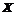- coordinate is a double root of the

polynomial. sinceis of degree 3, this gives us only three possibilities:

·has no multiple roots, and the equation defines an elliptic curve (Three distinct roots),  (For example, elliptic curve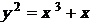has three distinct  roots).

·has a double root (curve has a node), (For example, curve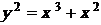has a node).

·has a triple root (curve has a cusp), (For example, curve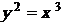has a cusp).

Attention. If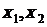and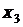are the roots of the polynomial, the discriminant for the equationturns out to be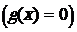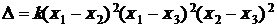where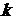is a constant.

This does just what we want:

If two of the roots are equal, it is Zero, and if not, not. Further more, it is not too hard to see that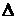is actually a polynomial in the coefficients of, which is what we

claimed. In other words, all that the discriminant is doing for us is giving a direct algebraic procedure for determining whether there are singular points.

while this analysis applies specifically to curves of the form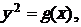it actually extends to all equations of the sort we are considering there is at most one singular point

and it is either a node or a cusp.

Attention. with some examples in hand, we can proceed to deeper waters. In order to understand the connection we are going to establish between elliptic curves and Fermat's

Last Theorem, we need to review quite a large portion of what is known about the rich arithmetic structure of these curves.

Conclusion. Elliptic curve of the form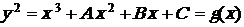is a elliptic curve of Non – Singular ifhas not a double root or

a triple root. In fact below equation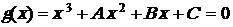has three distinct roots, if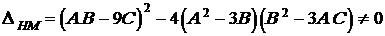Attention. If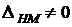thenhas no multiple roots, andis a

Non –Singular cubic elliptic curve.

###3 New Proof of Fermat's last Theorem by HM Final Main Theoremby

·       HM Final – Main Theorem

For every odd number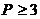Fermat's Last Theorem (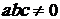):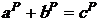Special case is of an elliptic curve (Non – Singular Cubic Curve) HM: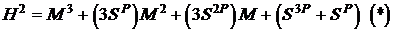·       Proof:

It is enough in the below general elliptic curve: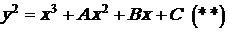Or elliptic curve HM (*) we assume: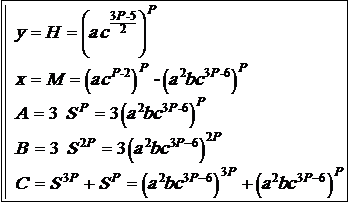then after replacing in (*) or (**):

(odd numbers)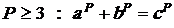Attention:

§  Elliptic curve (*) is Non – Singular.

§  First Fermat's equation is multiplied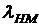.

§  We assume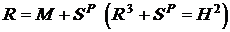.

§  We know that Proofed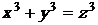is an elliptic curve.

New Proof of Fermat's Last Theorem by HM Final – Main Theorem

Because: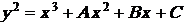;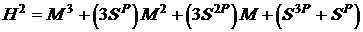;;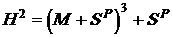;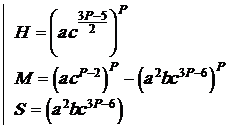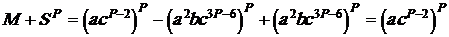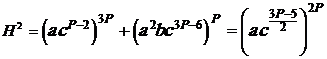;

(odd numbers);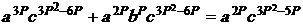;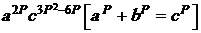;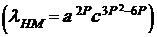;Attention:

§  Elliptic curve HM (*) is non – Singular, because: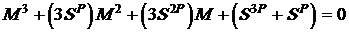;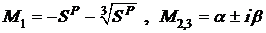(Three different roots)

·        References

 S. M. R. Hashemi Moosavi, The Discovery of Prime Numbers Formula & It's Results (2003). (ISBN: 978-600-94467-9-7).

 S. M. R. Hashemi Moosavi, Generalization of Fermat's Last Theorem and Solution of Beal's Equation by HM Theorems (2016). (ISBN: 978-600-94467-3-5).

 S. M. R. Hashemi Moosavi, 31 Methods for Solving Cubic Equations and Applications (2016). (ISBN: 978-600-94467-7-3).